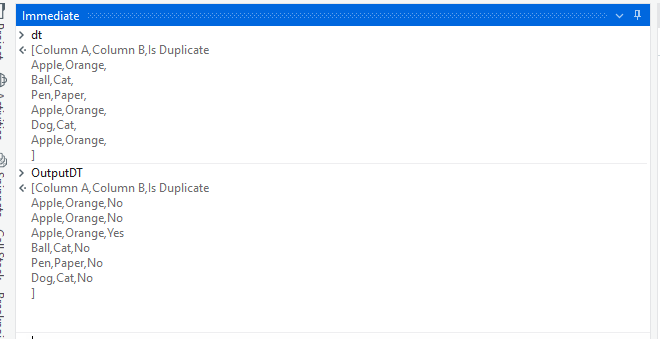# Linq Query for Marking duplicates as duplicates but leave the last record as Non Duplicate

Hi guys,

I need the linq query for below scenario.

In below image, I am taking out the duplicates base on the column A and Column B. So my row 2,3 and 7 are duplicate, So I want to mark 2 and 3 as duplicate and will leave the last occurring duplicate entry as non duplicate.

If not clear, then let’s see second example so, 4th and 9th rows are duplicate so I will mark only 4th row as duplicate and will leave the last occurring entry as non duplicate. So that I can process those records.

(Note: if there are duplicates then I want the last occurring entry mark as non duplicate. Also, Lets say if there is no duplicates and only one row entry then that entry will remain as non duplicate. Which you can check in 3rd row.)

1 Like

@shreyash_shirbhate

This was an interesting problem, check if the below works for you:

``````dt2 = dt.AsEnumerable()
.GroupBy(row => new
{
ColumnA = row.Field<string>("ColumnA"),
ColumnB = row.Field<string>("ColumnB")
})
.SelectMany(group =>
{
var lastIndex = group.Last();
group.ToList().ForEach(row => row.SetField("IsDuplicate", row == lastIndex ? "No" : "Yes"));
return group;
})
.CopyToDataTable();
``````

Here’s how my the workflow looks like:

Arguments to Invoke Code activity:

2 Likes

Thanks for the reply. You did not get any compilation error for Invoke code? Because I am getting below one. I used the same expression which you have provided.

Did you select the language as C#?

1 Like

@shreyash_shirbhate

Did that work? Let us know if you need any help!

1 Like

Hey Rahul, I will let you know. I am testing out few scenarios. @monsieurrahul

Thanks.

1 Like

If you can’t get a good LINQ query.

You can accomplish this in O(n) time&space by traversing from end->start of the datatable and using a dictionary<string,bool> to track occurences.

Could check with the below as well :

``````(From d In dt
Group d By k=d("Column A").toString.Trim,l=d("Column B").toString.Trim Into grp=Group
From g In grp.AsEnumerable
Let gra= If(grp.Count>1 AndAlso CType(g,DataRow).Equals(CType(grp.Last,DataRow)),g.ItemArray.Take(g.ItemArray.Count-1).Append("Yes").ToArray,g.ItemArray.Take(g.ItemArray.Count-1).Append("No").ToArray)
).CopyToDatatable
``````

From Debug Panel :Let us know if the ordering also matters, as we do see a change in the original data order.

2 Likes

When we can rely on the structure then we could do it with LINQ and DataTable reconstruction approach by

Assign Activity
dtResult = dtOrig.Clone

Assign Activity
dtResult =

``````(From d in dtOrig.AsEnumerable()
Group d by k1= d("ColumnsA").toString.Trim, k2= d("ColumnsB").toString.Trim into grp=Group
From i in Enumerable.Range(0,grp.Count)
Let g = grp(i)
Let isd = If(i = grp.Count -1, "No","Yes")
Let ra = g.ItemArray.Take(2).Append(isd).toArray